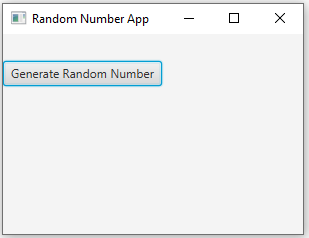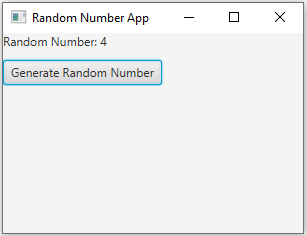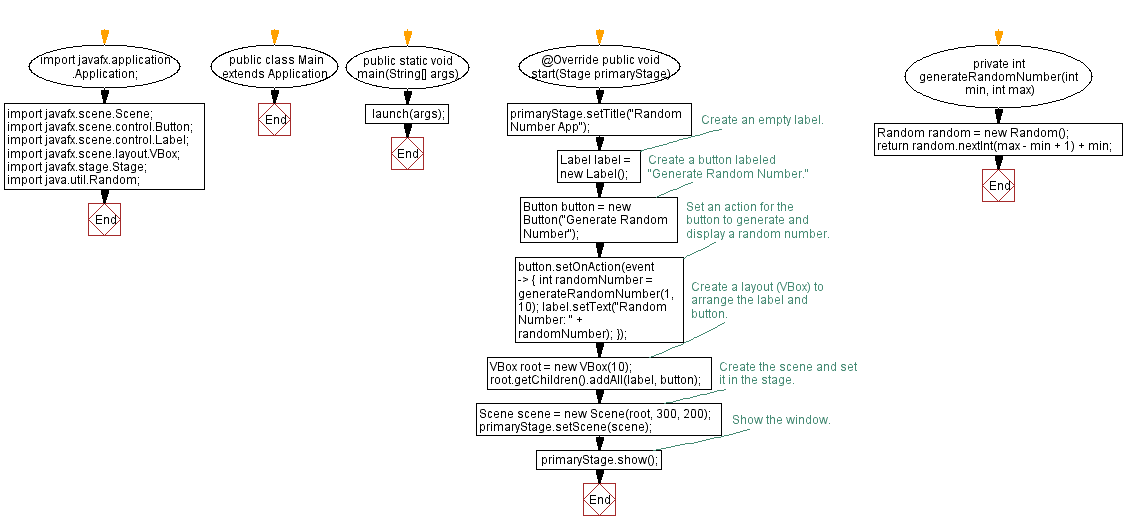﻿ JavaFX Random number generator

# JavaFX Random number generator

## JavaFx User Interface Components: Exercise-3 with Solution

Write a JavaFX application with a label and a button. Initially, the label should be empty. When the button is clicked, set the label text to a random number between 1 and 10.

Sample Solution:

JavaFx Code:

``````import javafx.application.Application;
import javafx.scene.Scene;
import javafx.scene.control.Button;
import javafx.scene.control.Label;
import javafx.scene.layout.VBox;
import javafx.stage.Stage;

import java.util.Random;

public class Main extends Application {

public static void main(String[] args) {
launch(args);
}

@Override
public void start(Stage primaryStage) {
primaryStage.setTitle("Random Number App");

// Create an empty label.
Label label = new Label();

// Create a button labeled "Generate Random Number."
Button button = new Button("Generate Random Number");

// Set an action for the button to generate and display a random number.
button.setOnAction(event -> {
int randomNumber = generateRandomNumber(1, 10);
label.setText("Random Number: " + randomNumber);
});

// Create a layout (VBox) to arrange the label and button.
VBox root = new VBox(10);

// Create the scene and set it in the stage.
Scene scene = new Scene(root, 300, 200);
primaryStage.setScene(scene);

// Show the window.
primaryStage.show();
}

private int generateRandomNumber(int min, int max) {
Random random = new Random();
return random.nextInt(max - min + 1) + min;
}
}
```
```

In the exercise above, we create an empty label and a button labeled "Generate Random Number." When you click the button, an action is triggered to generate a random number between 1 and 10 using the "generateRandomNumber()" method. The generated random number is then displayed in the label. The label, button, and layout are organized using a 'VBox'.

Sample Output:

``````
``````

Flowchart:Java Code Editor:

What is the difficulty level of this exercise?

Test your Programming skills with w3resource's quiz.

﻿